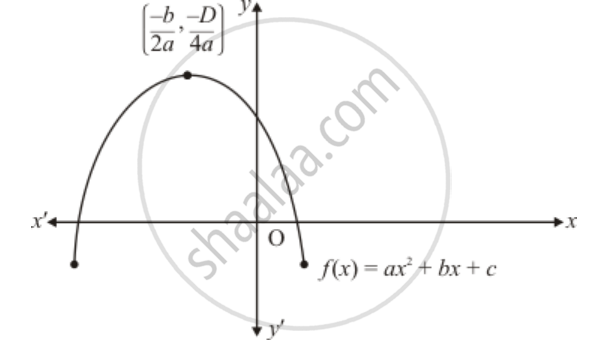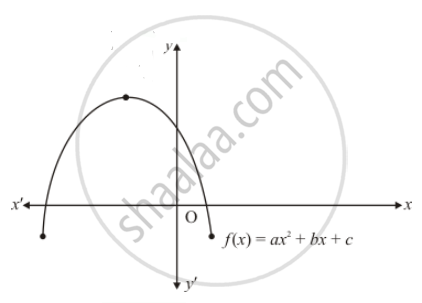Advertisement Remove all ads

# Figure 2.23 Show the Graph of the Polynomial F(X) = Ax2 + Bx + C for Which - Mathematics

MCQ

Figure 2.23 show the graph of the polynomial f(x) = ax2 + bx + c for which#### Options

• a < 0, b > 0 and c > 0

• a < 0, b < 0 and c > 0

• a < 0, b < 0 and c < 0

• a > 0, b > 0 and c < 0

Advertisement Remove all ads

#### Solution

Clearly, f(x) = ax2 + bx + c represent a parabola opening downwards. Therefore, a < 0

 y= ax^2 + bx + c   cuts y-axis at P which lies on OY. Putting x = 0 in  y = ax^2 + bx + c , we get y =c. So the coordinates P are (0,c). Clearly, P lies on (OY). Therefore  c > 0

The vertex  (-b)/(2a), (-D)/(4a) of the parabola is in the second quadrant. Therefore -b /(2a), b < 0Therefore a < 0,b>0 and c > 0

Hence, the correct choice is (b)

Is there an error in this question or solution?
Advertisement Remove all ads

#### APPEARS IN

RD Sharma Class 10 Maths
Chapter 2 Polynomials
Q 10 | Page 61
Advertisement Remove all ads

#### Video TutorialsVIEW ALL 

Advertisement Remove all ads
Share
Notifications

View all notifications

Forgot password?